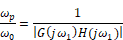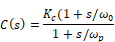### Lag compensator

The primary function of the lag compensator is to increase the low frequency gain to reduce steady-state errors and decrease sensitivity to noise and parameter variations.

To design a lag compensator

1.    Adjust the DC gain of the open-loop transfer function GH(s) to satisfy low-frequency specifications. Let the adjusted gain be Kc.

2.    Find the frequency ω1 for which the angle of G(jω) H(jω), θGH, is equal to (-180o + θM + 5o), where θM is the specified phase margin.

The magnitude of the lag zero is then equal to ω0 = 0.1 ω1.

3.    The ratio of the lag pole to lag zero is given by:4.    Solve the equations in steps 3 and 4 for ω0 and ωp.

5.    The compensator transfer function is then given by:This procedure is used to interactively design a lag compensator in the following section.